# 用Python实现BP神经网络（附代码）

大家好 我是毕加锁(锁!)

BP神经网络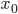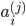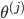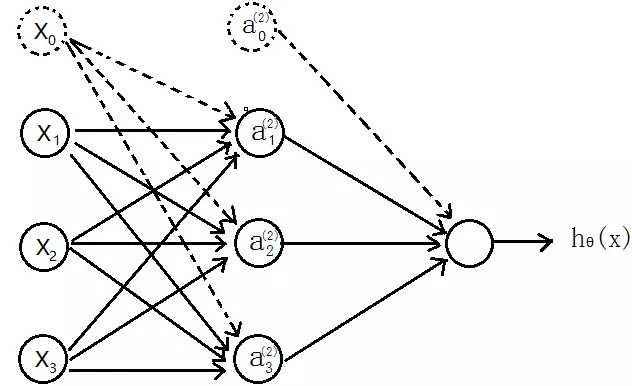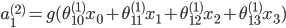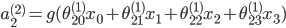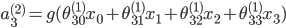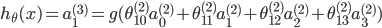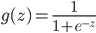，也成为激励函数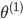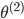==》j+1的单元数x（j层的单元数+1）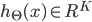，即代表输出层有K个单元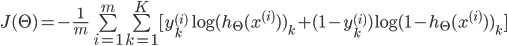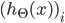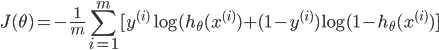L–>所有层的个数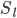–>第l层unit的个数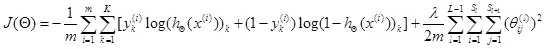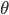1 2 3 4 5 6 7 8 9 10 11 12 13 14 15 16 17 18 19 20 21 22 23 24 25 26 27 28 29 30 31 32 33 34 35 36 37 38 39 40 41 42 43 44 45 46 47 48 49 50 51 52 53 54 55 56 57 `# 代价函数` `def` `nnCostFunction(nn_params,input_layer_size,hidden_layer_size,num_labels,X,y,Lambda):` `length ``=` `nn_params.shape[``0``] ``# theta的中长度` `# 还原theta1和theta2` `Theta1 ``=` `nn_params[``0``:hidden_layer_size``*``(input_layer_size``+``1``)].reshape(hidden_layer_size,input_layer_size``+``1``)` `Theta2 ``=` `nn_params[hidden_layer_size``*``(input_layer_size``+``1``):length].reshape(num_labels,hidden_layer_size``+``1``)` `# np.savetxt("Theta1.csv",Theta1,delimiter=',')` `m ``=` `X.shape[``0``]` `class_y ``=` `np.zeros((m,num_labels)) ``# 数据的y对应0-9，需要映射为0/1的关系` `# 映射y` `for` `i ``in` `range``(num_labels):` `class_y[:,i] ``=` `np.int32(y``=``=``i).reshape(``1``,``-``1``) ``# 注意reshape(1,-1)才可以赋值` `'''去掉theta1和theta2的第一列，因为正则化时从1开始'''` `Theta1_colCount ``=` `Theta1.shape[``1``]` `Theta1_x ``=` `Theta1[:,``1``:Theta1_colCount]` `Theta2_colCount ``=` `Theta2.shape[``1``]` `Theta2_x ``=` `Theta2[:,``1``:Theta2_colCount]` `# 正则化向theta^2` `term ``=` `np.dot(np.transpose(np.vstack((Theta1_x.reshape(``-``1``,``1``),Theta2_x.reshape(``-``1``,``1``)))),np.vstack((Theta1_x.reshape(``-``1``,``1``),Theta2_x.reshape(``-``1``,``1``))))` `'''正向传播,每次需要补上一列1的偏置bias'''` `a1 ``=` `np.hstack((np.ones((m,``1``)),X))` `z2 ``=` `np.dot(a1,np.transpose(Theta1))` `a2 ``=` `sigmoid(z2)` `a2 ``=` `np.hstack((np.ones((m,``1``)),a2))` `z3 ``=` `np.dot(a2,np.transpose(Theta2))` `h ``=` `sigmoid(z3)` `'''代价'''` `J ``=` `-``(np.dot(np.transpose(class_y.reshape(``-``1``,``1``)),np.log(h.reshape(``-``1``,``1``)))``+``np.dot(np.transpose(``1``-``class_y.reshape(``-``1``,``1``)),np.log(``1``-``h.reshape(``-``1``,``1``)))``-``Lambda``*``term``/``2``)``/``m` `return` `np.ravel(J)`

BP反向传播的目的就是求代价函数的梯度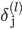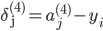《===》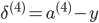（向量化）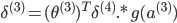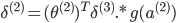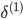，因为对于输入没有误差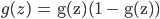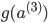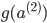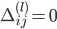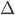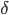for i=1-m:-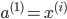-正向传播计算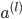（l=2,3,4…L）

-反向计算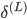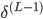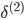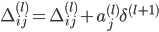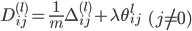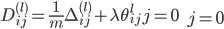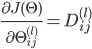，即得到代价函数的梯度

 1 2 3 4 5 6 7 8 9 10 11 12 13 14 15 16 17 18 19 20 21 22 23 24 25 26 27 28 29 30 31 32 33 34 35 36 37 38 39 40 41 42 43 44 45 46 47 48 49 50 51 52 53 54 55 56 57 58 59 60 61 62 63 64 65 66 67 68 69 70 71 72 73 `# 梯度` `def` `nnGradient(nn_params,input_layer_size,hidden_layer_size,num_labels,X,y,Lambda):` `length ``=` `nn_params.shape[``0``]` `Theta1 ``=` `nn_params[``0``:hidden_layer_size``*``(input_layer_size``+``1``)].reshape(hidden_layer_size,input_layer_size``+``1``)` `Theta2 ``=` `nn_params[hidden_layer_size``*``(input_layer_size``+``1``):length].reshape(num_labels,hidden_layer_size``+``1``)` `m ``=` `X.shape[``0``]` `class_y ``=` `np.zeros((m,num_labels)) ``# 数据的y对应0-9，需要映射为0/1的关系` `# 映射y` `for` `i ``in` `range``(num_labels):` `class_y[:,i] ``=` `np.int32(y``=``=``i).reshape(``1``,``-``1``) ``# 注意reshape(1,-1)才可以赋值` `'''去掉theta1和theta2的第一列，因为正则化时从1开始'''` `Theta1_colCount ``=` `Theta1.shape[``1``]` `Theta1_x ``=` `Theta1[:,``1``:Theta1_colCount]` `Theta2_colCount ``=` `Theta2.shape[``1``]` `Theta2_x ``=` `Theta2[:,``1``:Theta2_colCount]` `Theta1_grad ``=` `np.zeros((Theta1.shape)) ``#第一层到第二层的权重` `Theta2_grad ``=` `np.zeros((Theta2.shape)) ``#第二层到第三层的权重` `Theta1[:,``0``] ``=` `0``;` `Theta2[:,``0``] ``=` `0``;` `'''正向传播，每次需要补上一列1的偏置bias'''` `a1 ``=` `np.hstack((np.ones((m,``1``)),X))` `z2 ``=` `np.dot(a1,np.transpose(Theta1))` `a2 ``=` `sigmoid(z2)` `a2 ``=` `np.hstack((np.ones((m,``1``)),a2))` `z3 ``=` `np.dot(a2,np.transpose(Theta2))` `h ``=` `sigmoid(z3)` `'''反向传播，delta为误差，'''` `delta3 ``=` `np.zeros((m,num_labels))` `delta2 ``=` `np.zeros((m,hidden_layer_size))` `for` `i ``in` `range``(m):` `delta3[i,:] ``=` `h[i,:]``-``class_y[i,:]` `Theta2_grad ``=` `Theta2_grad``+``np.dot(np.transpose(delta3[i,:].reshape(``1``,``-``1``)),a2[i,:].reshape(``1``,``-``1``))` `delta2[i,:] ``=` `np.dot(delta3[i,:].reshape(``1``,``-``1``),Theta2_x)``*``sigmoidGradient(z2[i,:])` `Theta1_grad ``=` `Theta1_grad``+``np.dot(np.transpose(delta2[i,:].reshape(``1``,``-``1``)),a1[i,:].reshape(``1``,``-``1``))` `'''梯度'''` `grad ``=` `(np.vstack((Theta1_grad.reshape(``-``1``,``1``),Theta2_grad.reshape(``-``1``,``1``)))``+``Lambda``*``np.vstack((Theta1.reshape(``-``1``,``1``),Theta2.reshape(``-``1``,``1``))))``/``m` `return` `np.ravel(grad)`

BP可以求梯度的原因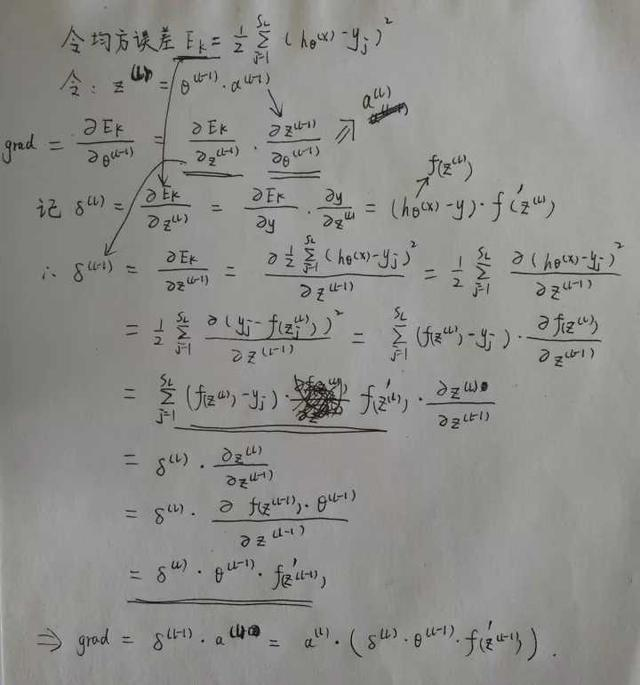字比较草 勿喷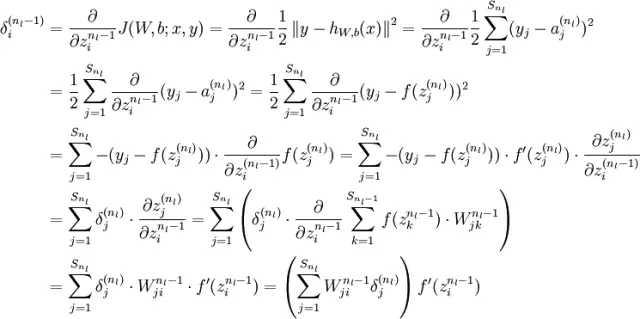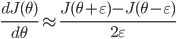1 2 3 4 5 6 7 8 9 10 11 12 13 14 15 16 17 18 19 20 21 22 23 24 25 26 27 28 29 30 31 32 33 34 35 36 37 38 39 40 41 42 43 44 45 46 47 48 49 50 51 52 53 54 55 56 57 58 59 60 61 62 63 64 65 66 67 `# 检验梯度是否计算正确` `# 检验梯度是否计算正确` `def` `checkGradient(Lambda ``=` `0``):` `'''构造一个小型的神经网络验证，因为数值法计算梯度很浪费时间，而且验证正确后之后就不再需要验证了'''` `input_layer_size ``=` `3` `hidden_layer_size ``=` `5` `num_labels ``=` `3` `m ``=` `5` `initial_Theta1 ``=` `debugInitializeWeights(input_layer_size,hidden_layer_size);` `initial_Theta2 ``=` `debugInitializeWeights(hidden_layer_size,num_labels)` `X ``=` `debugInitializeWeights(input_layer_size``-``1``,m)` `y ``=` `1``+``np.transpose(np.mod(np.arange(``1``,m``+``1``), num_labels))``# 初始化y` `y ``=` `y.reshape(``-``1``,``1``)` `nn_params ``=` `np.vstack((initial_Theta1.reshape(``-``1``,``1``),initial_Theta2.reshape(``-``1``,``1``))) ``#展开theta` `'''BP求出梯度'''` `grad ``=` `nnGradient(nn_params, input_layer_size, hidden_layer_size,` `num_labels, X, y, Lambda)` `'''使用数值法计算梯度'''` `num_grad ``=` `np.zeros((nn_params.shape[``0``]))` `step ``=` `np.zeros((nn_params.shape[``0``]))` `e ``=` `1e``-``4` `for` `i ``in` `range``(nn_params.shape[``0``]):` `step[i] ``=` `e` `loss1 ``=` `nnCostFunction(nn_params``-``step.reshape(``-``1``,``1``), input_layer_size, hidden_layer_size,` `num_labels, X, y,` `Lambda)` `loss2 ``=` `nnCostFunction(nn_params``+``step.reshape(``-``1``,``1``), input_layer_size, hidden_layer_size,` `num_labels, X, y,` `Lambda)` `num_grad[i] ``=` `(loss2``-``loss1)``/``(``2``*``e)` `step[i]``=``0` `# 显示两列比较` `res ``=` `np.hstack((num_grad.reshape(``-``1``,``1``),grad.reshape(``-``1``,``1``)))` `print` `res`

 1 2 3 4 5 6 7 8 9 10 11 `# 随机初始化权重theta` `def` `randInitializeWeights(L_in,L_out):` `W ``=` `np.zeros((L_out,``1``+``L_in)) ``# 对应theta的权重` `epsilon_init ``=` `(``6.0``/``(L_out``+``L_in))``*``*``0.5` `W ``=` `np.random.rand(L_out,``1``+``L_in)``*``2``*``epsilon_init``-``epsilon_init ``# np.random.rand(L_out,1+L_in)产生L_out*(1+L_in)大小的随机矩阵` `return` `W`

 1 2 3 4 5 6 7 8 9 10 11 12 13 14 15 16 17 18 19 20 21 22 23 24 25 26 27 28 29 30 31 32 33 34 35 36 37 38 39 40 41 `# 预测` `def` `predict(Theta1,Theta2,X):` `m ``=` `X.shape[``0``]` `num_labels ``=` `Theta2.shape[``0``]` `#p = np.zeros((m,1))` `'''正向传播，预测结果'''` `X ``=` `np.hstack((np.ones((m,``1``)),X))` `h1 ``=` `sigmoid(np.dot(X,np.transpose(Theta1)))` `h1 ``=` `np.hstack((np.ones((m,``1``)),h1))` `h2 ``=` `sigmoid(np.dot(h1,np.transpose(Theta2)))` `'''` `返回h中每一行最大值所在的列号` `- np.max(h, axis=1)返回h中每一行的最大值（是某个数字的最大概率）` `- 最后where找到的最大概率所在的列号（列号即是对应的数字）` `'''` `#np.savetxt("h2.csv",h2,delimiter=',')` `p ``=` `np.array(np.where(h2[``0``,:] ``=``=` `np.``max``(h2, axis``=``1``)[``0``]))` `for` `i ``in` `np.arange(``1``, m):` `t ``=` `np.array(np.where(h2[i,:] ``=``=` `np.``max``(h2, axis``=``1``)[i]))` `p ``=` `np.vstack((p,t))` `return` `p`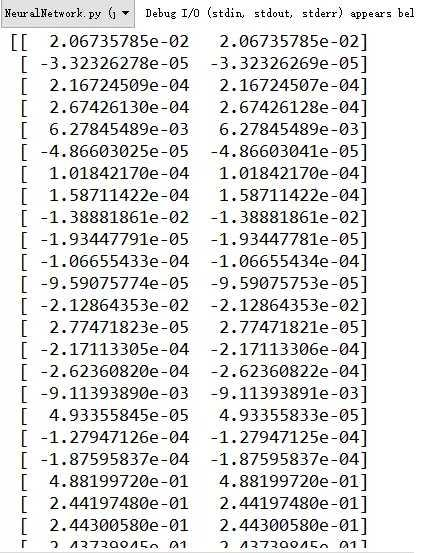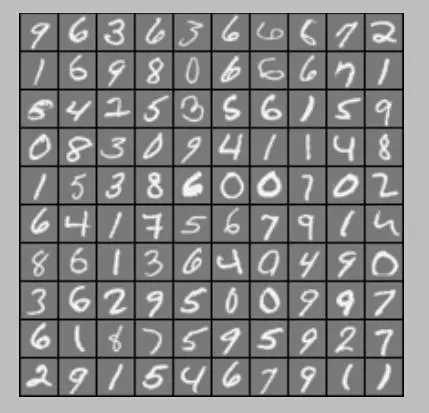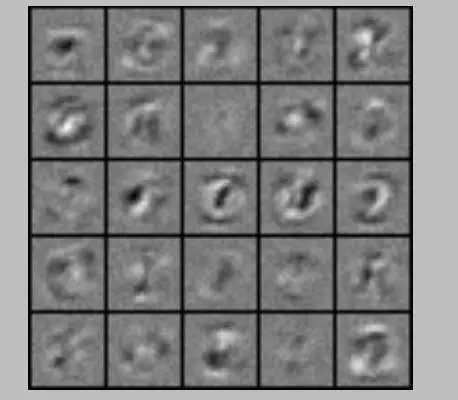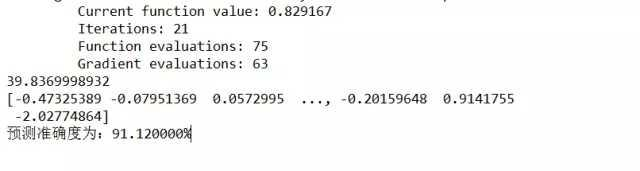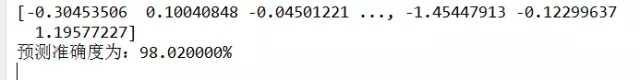我是毕加锁 期待你的关注